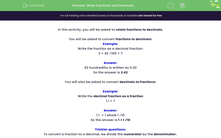# Write Fractions and Decimals

In this worksheet, students will be asked to relate fractions to decimal equivalents.Key stage:  KS 2

Curriculum topic:   Number: Fractions, Decimals and Percentages

Curriculum subtopic:   Relate Fractions to Decimals

Difficulty level:#### Worksheet Overview

In this activity, you will be asked to relate fractions to decimals.

You will be asked to convert fractions to decimals:

Example:

Write the fraction as a decimal:

2 + 42 ⁄100 = ?

42 hundredths is written as 0.42

You will also be asked to convert decimals to fractions:

Example:

Write the decimal as a fraction

1.1 = ?

1.1  = 1 whole 1 ⁄10

So the answer is 1 + 1 ⁄10

Trickier questions:

To convert a fraction to a decimal, we divide the numerator by the denominator.

1 ⁄2 = 1 ÷ 2 = 0.5

Sometimes it helps to use a calculator.

1 ⁄4 = 0.25

1 ⁄3 = 0.3

1 ⁄6 = 0.16

1 ⁄8 = 0.125

1⁄10 = 0.1

1 ⁄5 = 0.2

### What is EdPlace?

We're your National Curriculum aligned online education content provider helping each child succeed in English, maths and science from year 1 to GCSE. With an EdPlace account you’ll be able to track and measure progress, helping each child achieve their best. We build confidence and attainment by personalising each child’s learning at a level that suits them.

Get started